# Writing ShapeSheet Formulas

3 4

ShapeSheet cells can contain either a value or a formula, and you can alternate the view to show one or the other. A formula is the expression in a cell that evaluates to a value. Visio adds some default formulas to shapes; a shape inherits other formulas from a master shape. And then there are the formulas that you can create by typing in a ShapeSheet cell or on the formula bar, as Figure 25-3 shows. A formula always starts with an equal sign, which Visio inserts automatically.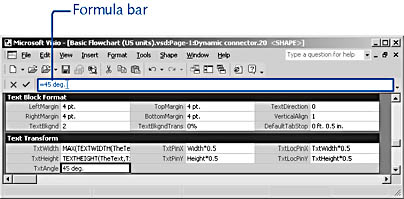Figure 25-3.  When you select a cell, its formula is shown on the formula bar. You can type directly in cells or use the formula bar to enter and edit formulas.

When you edit a cell's formula, you change the way that Visio calculates the value of the cell. The result is that shape behavior changes. For example, you can edit the formula in the TxtAngle cell of the Text Transform section by typing 45 deg. and then pressing Enter. The result is that the angle of the shape's text block is rotated 45 degrees on the drawing page. You can accomplish the same feat with the Text Block tool, so what's the difference? Editing formulas becomes much more interesting when you realize that you can calculate a shape's geometry or appearance dynamically. That is, you can write formulas to adjust the value of one cell based on the value of another. This feature is the heart of SmartShapes programming.

InsideOut

When you draw a shape, Visio creates default formulas for the shape. To see what default formulas look like, draw a simple shape (such as a rectangle, ellipse, or straight line), and then take a look at its ShapeSheet.

## Contents of a Formula

A Visio formula can contain the following elements:

• Coordinates. Most formulas are expressed as coordinates for controlling the position and size of a shape. A coordinate describes the position of a vertex with respect to the origin of a shape and to the origin of the group or page that contains the shape.
• Numbers. In a formula, numbers specify a wide range of values, such as measurements and angles. Constants are numbers that map to predefined Visio settings, such as those used to represent styles and colors. A number can include a plus (+) or a minus (–) sign and can be entered in exponential notation (for example, 1.2E-6) or as a fraction (for example, 5 9/16).
• Units of measure. Because many values in Visio are dimensional (for example, width in inches or angle in degrees), many formulas include measurement units after a number. If you don't specify a unit of measure, Visio uses default units of measure in cells that require a unit of measure.
• Cell references. A formula can contain the name of another cell, the value of which is used in the formula.
• Functions. Like Excel functions, Visio functions perform a task. Visio includes mathematical, trigonometric, logical, date and time, statistical, and other functions.
• Operators and parentheses. Formulas typically include mathematical operators (+ for addition, – for subtraction, * for multiplication, / for division) and parentheses that control the order of operation. Formulas can also contain the Boolean expressions TRUE and FALSE.
• Strings. A string is simply text in a formula usually set off with quotation marks.

For example, the default formula that Visio enters for the position of a shape's pin (its center of rotation) is stored in the LocPinX and LocPinY cells and looks like this:

` LocPinX=Width*0.5LocPinY=Height*0.5 `

### Learning to Write SmartShapes Formulas

The Microsoft Developer Network (MSDN) Web site includes resources for shape designers and programmers. At http://msdn.microsoft.com/visio, you'll find articles about SmartShapes programming as well as the text of the Developing Microsoft Visio Solutions programming guide, sample code, and information about training resources. If you want to learn more about SmartShapes programming and using other programming languages to extend Visio's functionality, the instructor-led training courses in Visio development are well worthwhile. Visio is an idiosyncratic program, and its development environment is quirky but very powerful. Visio is a full-fledged development platform that organizations have used to create some pretty amazing applications. The example applications on the MSDN Web site will show you some of the possibilities.

What does this tell you? In the top formula, the LocPinX cell represents the x-coordinate of the pin—that is, its horizontal location. Width is a cell reference and refers to the Width cell in the Shape Transform section and defines a measurement, such as 1 inch. The asterisk (*) symbol is the operator for multiplication. So, the horizontal location of the pin is the point that is half the value of the Width cell. In short, it's in the middle. The formula in the LocPinY cell is similar but defines the vertical distance of the pin in terms of the shape's height. Suppose you select the Rotation tool on the drawing page and use it to move the pin, thereby changing the shape's center of rotation. Visio writes new formulas into these cells to describe the pin's new position.

## Creating and Editing Formulas

To create a formula, you can select a cell and type, just as you can select a shape and type. Similarly, you can double-click a cell to display the insertion point so that you can edit a formula. You can also use the formula bar, which works much like the one in Excel. It's somewhat easier to type on the formula bar, because you can see more of your formula at once.

Note

If you click a cell and type, you overwrite the existing formula entirely. To add to or edit the formula in a cell, double-click the cell or select it, and then press F2.

Follow these steps to type a new formula for a cell:

1. In the ShapeSheet window, click a cell to select it and display its formula on the formula bar.2. Type the formula.
3. To accept the formula, click the Accept button (the blue check mark) on the formula bar or press Enter. If the number or formula contains an error, Visio displays a message.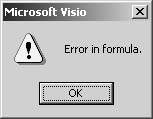4. In the message box, click OK. Visio highlights the error on the formula bar.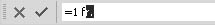5. Correct the error, and then click Accept or press Enter.

Tip

When you're typing in a cell or on the formula bar, you can cancel your changes by clicking the Cancel Change button (the dark red X) on the formula bar or by pressing the Esc key.

You can edit formulas on the formula bar, much as you do in a spreadsheet. Table 25-3 describes how.

Table 25-3. Techniques for Editing on the Formula Bar

Place the insertion point.

Click where you want the insertion point to appear.

Move the insertion point.

Press the Left or Right Arrow key.

Delete the character to the left.

Press Backspace.

Delete the character to the right.

Press Delete.

Select text.

Drag the mouse over the text.

Extend or reduce the selection to the left.

Press Shift+Left Arrow.

Extend or reduce the selection to the right.

Press Shift+Right Arrow.

Select a word.

Press Ctrl+Shift+Right Arrow or double-click.

Select to the end.

Press Shift+End.

Select to the beginning.

Press Shift+Home.

## Creating a Cell Reference in a Formula

Cell references provide a powerful way to create interdependent behavior in a shape. You can set one cell to a particular value based on the setting in another cell—in the same ShapeSheet or in the ShapeSheet for another object, including the page. You must follow Visio's naming syntax, which describes the rules for correctly referring to another cell. It's easy to refer to another cell in the same ShapeSheet. When you're typing a formula, you can click in another cell to add a reference to that cell in the formula, as Figure 25-4 shows.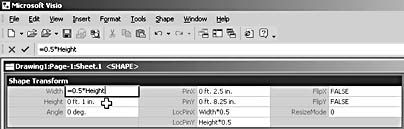Figure 25-4.  A reference to a cell in the same ShapeSheet specifies only the cell name, such as Height.

It gets a little trickier when you want to refer to another object's ShapeSheet cell. Then you must know the object's identifier or name and use an exclamation point (!) to separate the object name from the name of the cell like this:

` Sheet.12!Width `

Visio displays an object's identifier or name on the title bar of the ShapeSheet window, but you can also select an object, and then choose Format, Special, to display the identifier or name. An object's identifier, such as Sheet.12, doesn't change unless you move the object to another page or document.

Note

For more information about cell references, choose Help, Developer Reference. Click Contents. Expand Microsoft Visio Developer Reference, expand ShapeSheet Reference, expand Conceptual Information, and then click About Cell References.

## Entering Functions

You can perform a variety of calculations by using a function. Functions are really just predefined formulas that use specific values, called arguments, in a particular order. For example, the SUM function adds a series of numbers, and the UPPER function transforms text into uppercase letters. The structure of a function (that is, its syntax) requires you to use parentheses around the arguments. For example, the GOTOPAGE function uses the following syntax:

` GOTOPAGE(pagename) `

The GOTOPAGE function creates a link to another page specified by the pagename argument. You can use the name that appears on the page tabs at the bottom of the drawing page window. Because the page name is a string, you must enclose it in quotation marks. A valid formula using the GOTOPAGE function would look like this:

` GOTOPAGE("page-2") `

Follow these steps to quickly add a function to a ShapeSheet formula:

1. Double-click the cell, and then click where you want to insert the function.
2. Choose Insert, Function.3. Scroll the Select Function list, if necessary, and then select a function.
4. Select the Insert Arguments Into Formula Bar check box, and then click OK.
5. In the cell, type over the argument placeholders to insert the arguments you want to use, and then click the Accept button (the blue check mark) or press Enter to enter the formula.

Tip

You can press the Esc key to cancel the changes when you edit a formula.

## Protecting Shape Formulas

In other chapters, Visio's built-in protections have been discussed—features such as protection locks and other safeguards that prevent you from making changes to shapes. The equivalent mechanism in a ShapeSheet formula is the GUARD function, which prevents an expression from being overwritten by changes that occur on the drawing page. For example, when you click the Fill Color button and apply a new color to a shape, Visio records your action by changing the formula in the FillForegnd cell. If you want to prevent a shape's fill color from changing, you can guard the formula in that cell with the GUARD function. Many Visio shapes are guarded in this way to prevent SmartShapes formulas from being inadvertently changed. The syntax for the GUARD function looks like this:

` GUARD(expression) `

You most often see the GUARD function used in the Width, Height, PinX, and PinY cells of the Shape Transform section in Visio shapes. For example, when you drag a shape from the Backgrounds stencil, Visio sizes the background to fit the drawing page and guards the formulas that control size and position, as Figure 25-5 shows. The width and height of the background shape is set to match the page's width and height. The PinX, PinY, FlipX, and FlipY cells use the GUARD function to prevent the shape from being repositioned or flipped.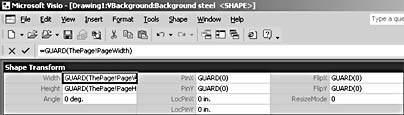Figure 25-5.  The GUARD functions in these formulas prevent the expressions from being replaced with other values. In effect, you cannot change the shape's width, height, position, or orientation on the drawing page.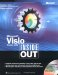Microsoft Visio Version 2002 Inside Out (Inside Out (Microsoft))
ISBN: 0735612854
EAN: 2147483647
Year: 2000
Pages: 211
Authors: Nanette Eaton